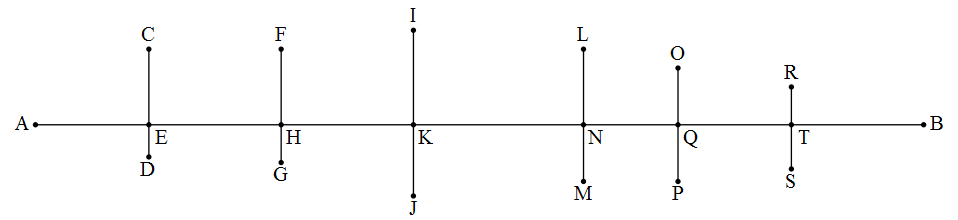# How do you know this is real?Which of the following statements are wrong?

• (a) Each point on the real line corresponds to a unique real number.
• (b) There are more real numbers between any two consecutive integers than the whole set of integers.
• (c) We can't say (b) above since we can't compare two infinities.
• (d) Every bounded interval is a finite set.
• (e) Some bounded intervals are finite sets.
• (f) There are finite irrational numbers between any two given irrational numbers.
×